Checking if funciton is differentiable

Chapter 5 Class 12 Continuity and Differentiability
Concept wise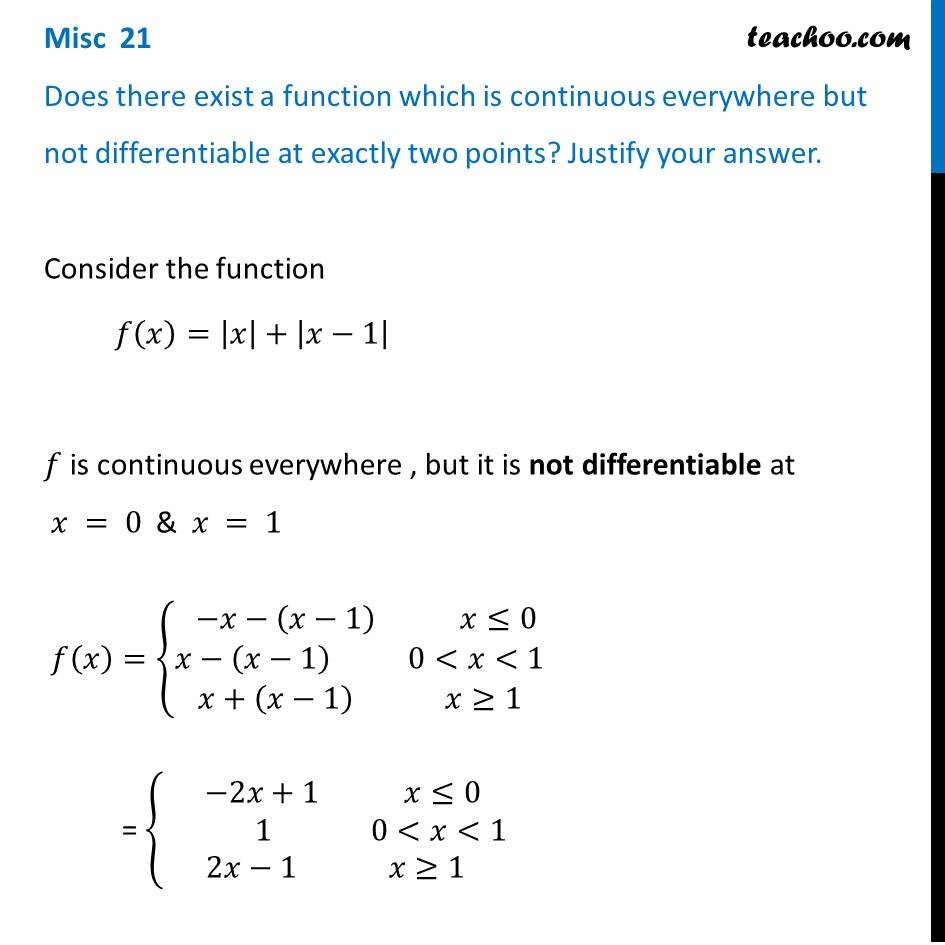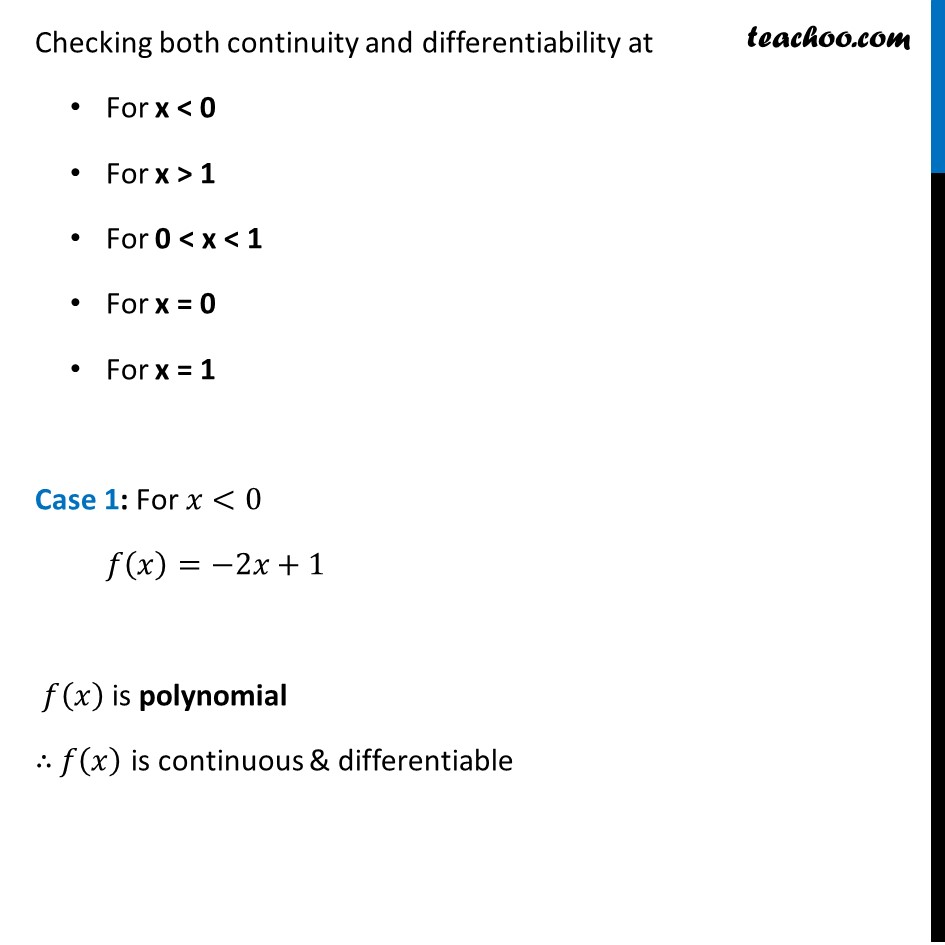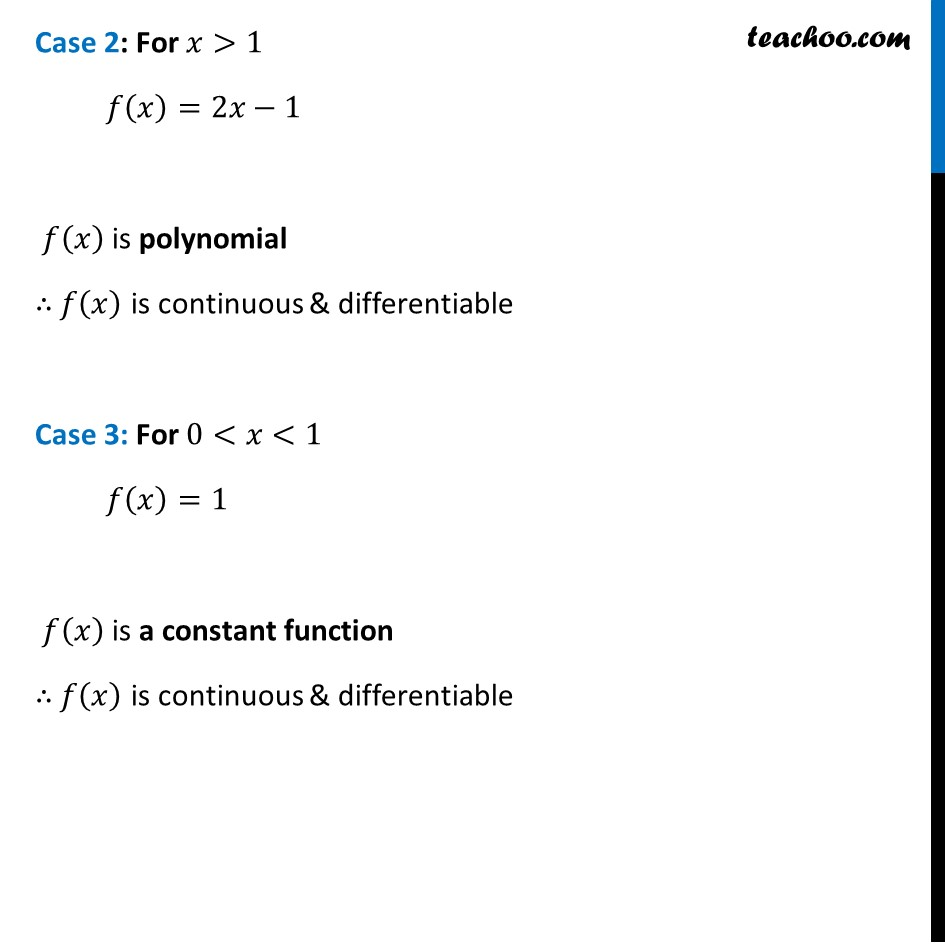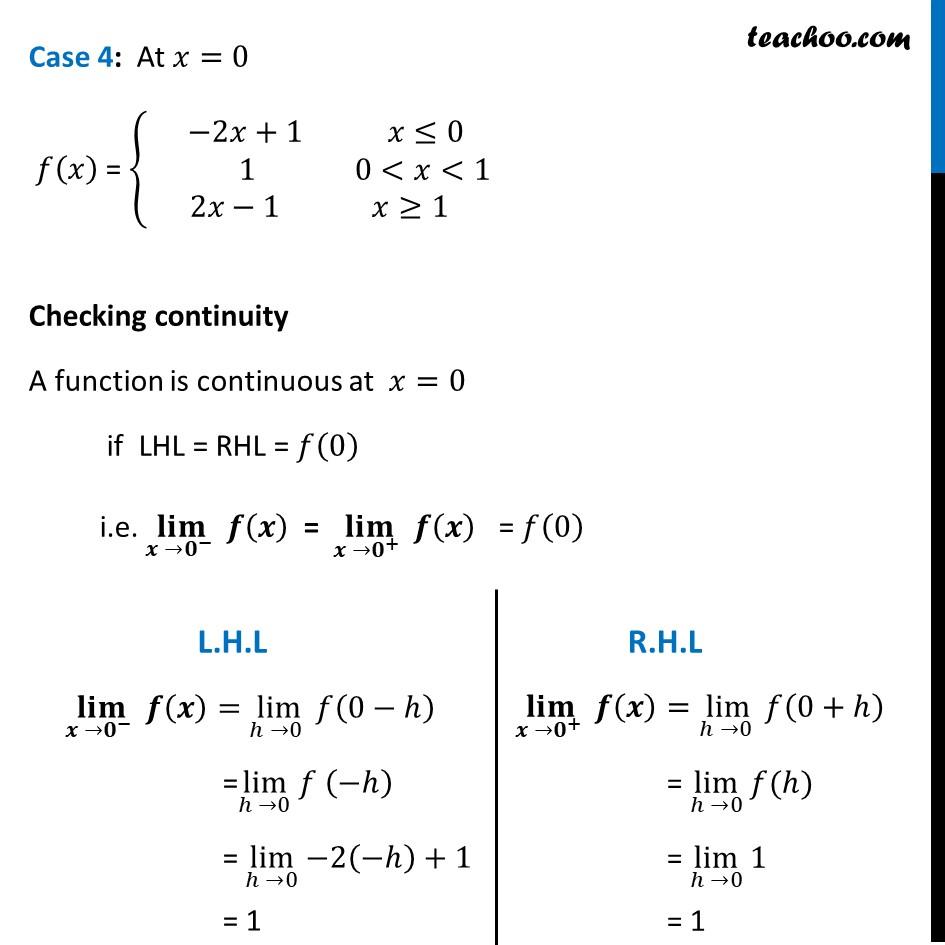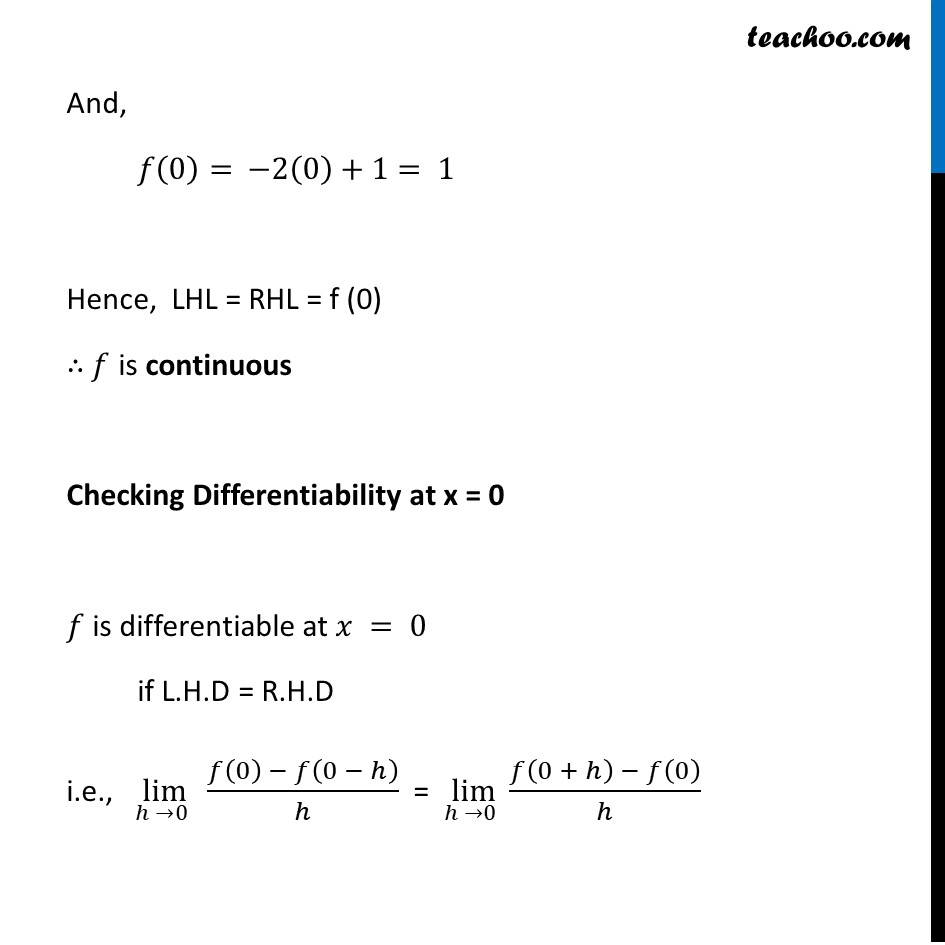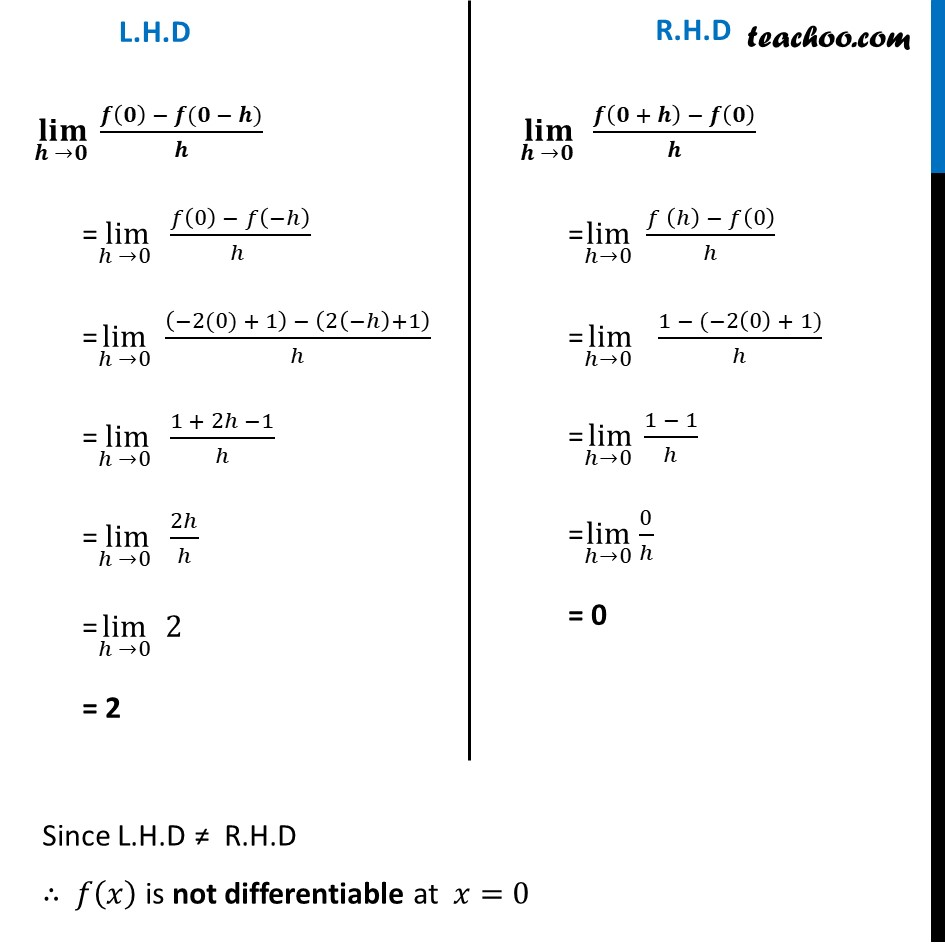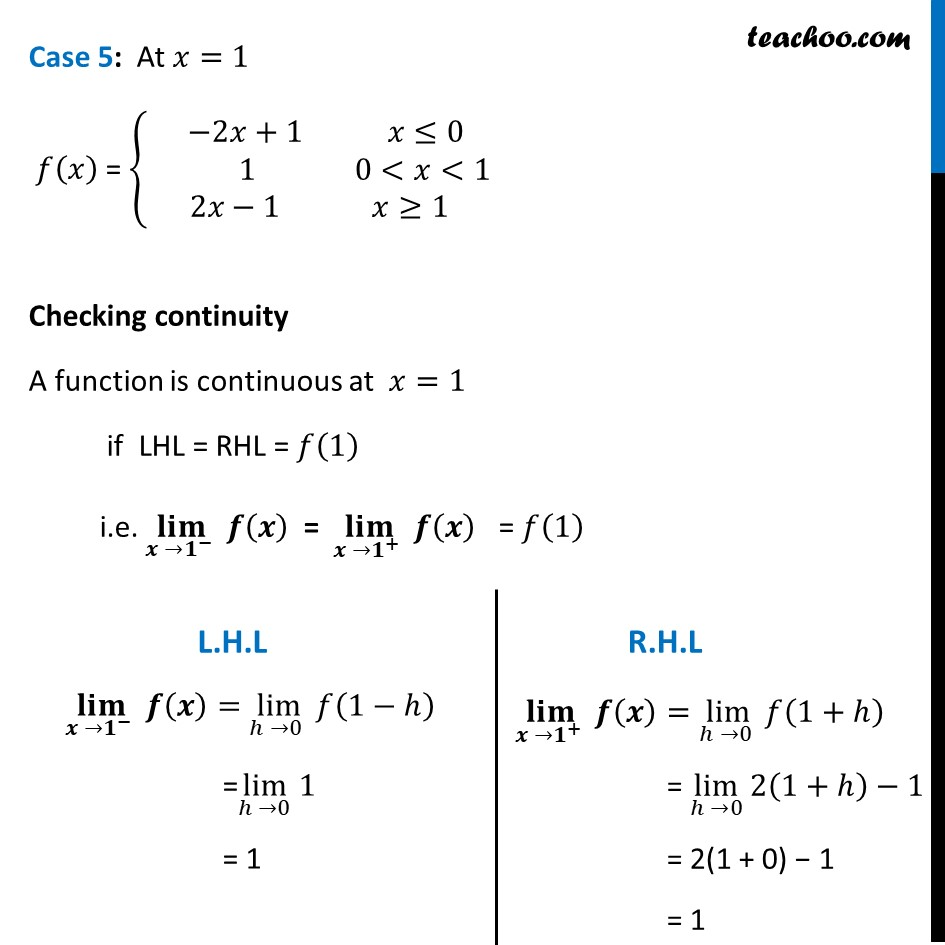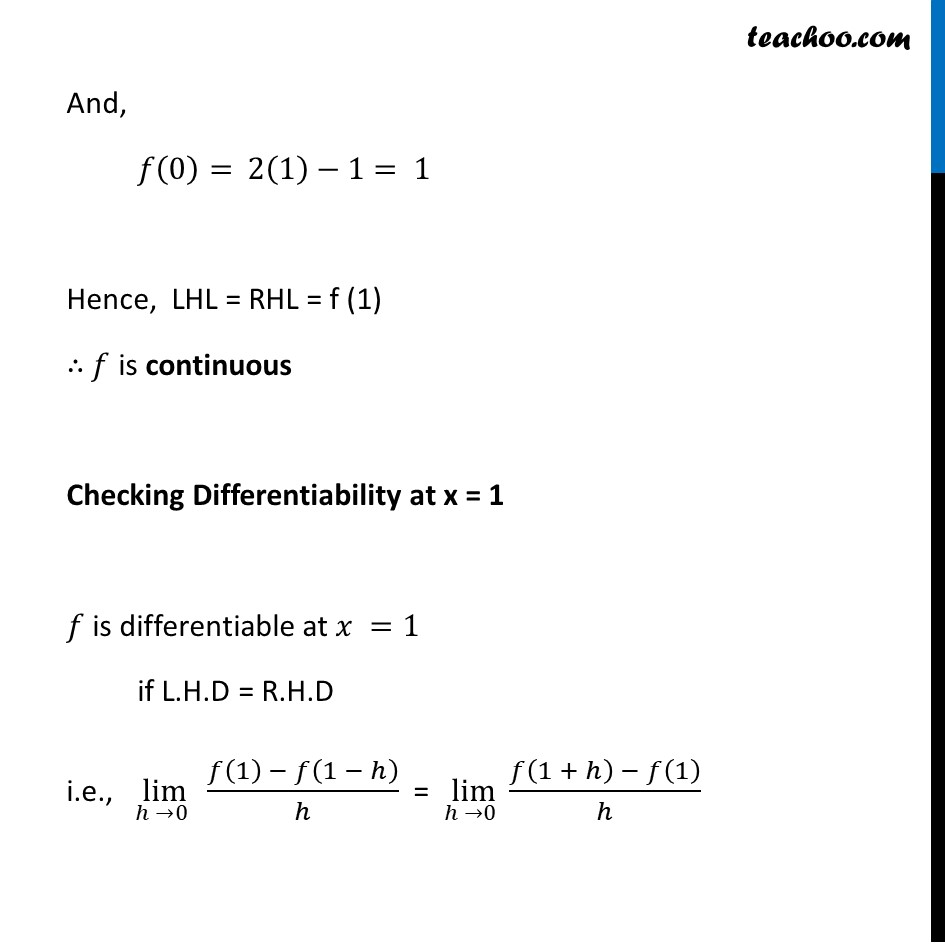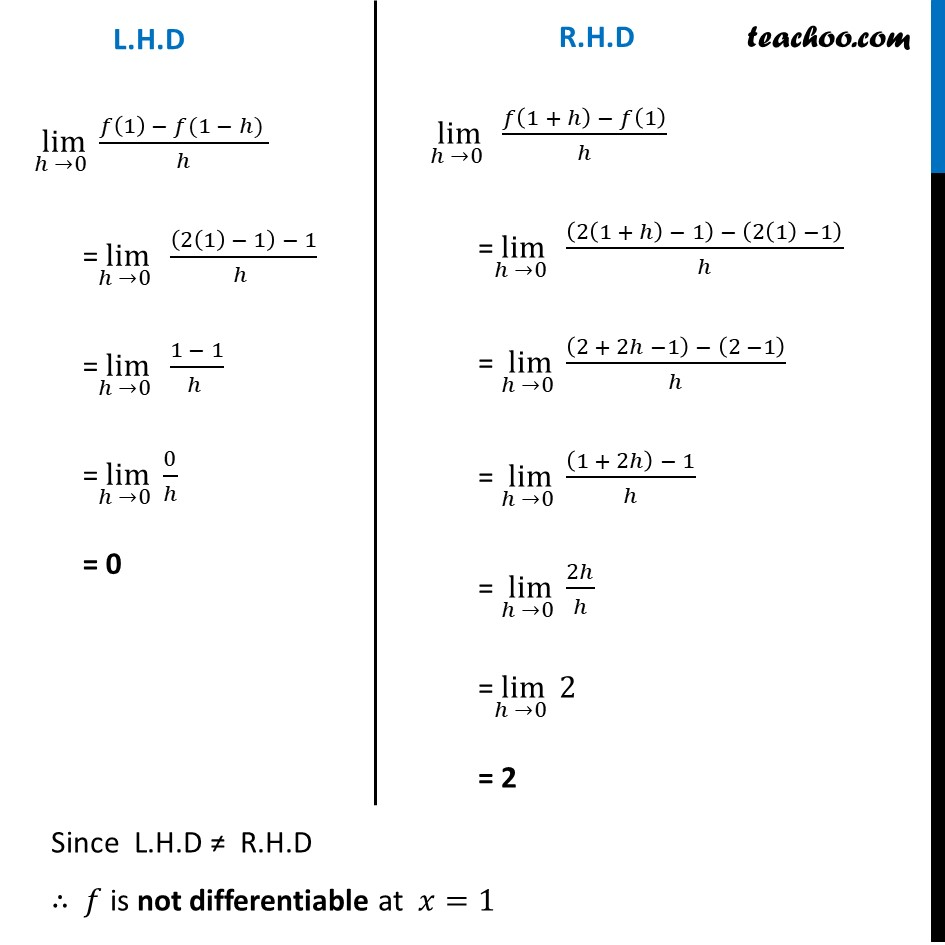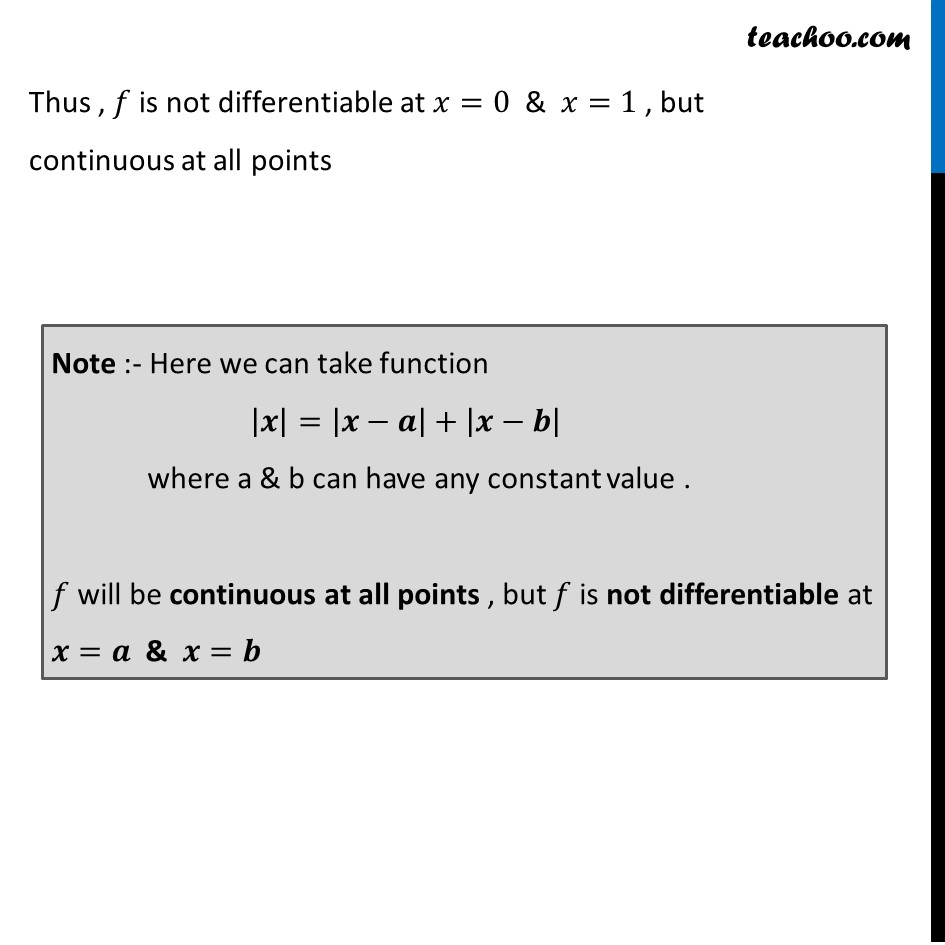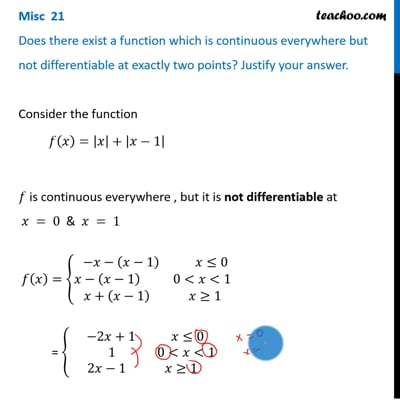This video is only available for Teachoo black users

Introducing your new favourite teacher - Teachoo Black, at only ₹83 per month

### Transcript

Misc 21 Does there exist a function which is continuous everywhere but not differentiable at exactly two points? Justify your answer.Consider the function 𝑓(𝑥)=|𝑥|+|𝑥−1| 𝑓 is continuous everywhere , but it is not differentiable at 𝑥 = 0 & 𝑥 = 1 𝑓(𝑥)={█( −𝑥−(𝑥−1) 𝑥≤0@𝑥−(𝑥−1) 0<𝑥<1@𝑥+(𝑥−1) 𝑥≥1)┤ = {█( −2𝑥+1 𝑥≤0@ 1 0<𝑥<1@2𝑥−1 𝑥≥1)┤ Checking both continuity and differentiability at For x < 0 For x > 1 For 0 < x < 1 For x = 0 For x = 1 Case 1: For 𝑥<0 𝑓(𝑥)=−2𝑥+1 𝑓(𝑥) is polynomial ∴ 𝑓(𝑥) is continuous & differentiable Case 2: For 𝑥>1 𝑓(𝑥)=2𝑥−1 𝑓(𝑥) is polynomial ∴ 𝑓(𝑥) is continuous & differentiable Case 3: For 0<𝑥<1 𝑓(𝑥)=1 𝑓(𝑥) is a constant function ∴ 𝑓(𝑥) is continuous & differentiable Case 4: At 𝑥=0 𝑓(𝑥) = {█( −2𝑥+1 𝑥≤0@ 1 0<𝑥<1@2𝑥−1 𝑥≥1)┤ Checking continuity A function is continuous at 𝑥=0 if LHL = RHL = 𝑓(0) i.e. (𝐥𝐢𝐦)┬(𝒙 →𝟎^− ) 𝒇(𝒙) = (𝐥𝐢𝐦)┬(𝒙 →𝟎^+ ) 𝒇(𝒙) = 𝑓(0) (𝐥𝐢𝐦)┬(𝒙 →𝟎^− ) 𝒇(𝒙)=lim┬(ℎ →0 ) 𝑓(0−ℎ) =lim┬(ℎ →0) 𝑓 (−ℎ) = lim┬(ℎ →0) −2(−ℎ)+1 = 1 (𝐥𝐢𝐦)┬(𝒙 →𝟎^+ ) 𝒇(𝒙)=lim┬(ℎ →0 ) 𝑓(0+ℎ) = lim┬(ℎ →0) 𝑓(ℎ) = lim┬(ℎ →0) 1 = 1 And, 𝑓(0)= −2(0)+1= 1 Hence, LHL = RHL = f (0) ∴ 𝑓 is continuous Checking Differentiability at x = 0 𝑓 is differentiable at 𝑥 = 0 if L.H.D = R.H.D i.e., lim┬(ℎ →0 ) (𝑓(0) − 𝑓(0 − ℎ))/ℎ = lim┬(ℎ →0 ) (𝑓(0 + ℎ) − 𝑓(0))/ℎ (𝐥𝐢𝐦)┬(𝒉 →𝟎 ) (𝒇(𝟎) − 𝒇(𝟎 − 𝒉))/𝒉 =lim┬(ℎ →0 ) (𝑓(0) − 𝑓(−ℎ))/(ℎ ) =lim┬(ℎ →0) ((−2(0) + 1) − (2(−ℎ)+1))/ℎ =lim┬(ℎ →0 ) (1 + 2ℎ −1)/ℎ =lim┬(ℎ →0 ) 2ℎ/ℎ =lim┬(ℎ →0) 2 = 2 (𝐥𝐢𝐦)┬(𝒉 →𝟎 ) (𝒇(𝟎 + 𝒉) − 𝒇(𝟎))/𝒉 =lim┬(ℎ→0) (𝑓 (ℎ) − 𝑓(0))/ℎ =lim┬(ℎ→0 ) (1 − (−2(0) + 1))/ℎ =lim┬(ℎ→0 ) (1 − 1)/ℎ =lim┬(ℎ→0) 0/ℎ = 0 Since L.H.D ≠ R.H.D ∴ 𝑓(𝑥) is not differentiable at 𝑥=0 Case 5: At 𝑥=1 𝑓(𝑥) = {█( −2𝑥+1 𝑥≤0@ 1 0<𝑥<1@2𝑥−1 𝑥≥1)┤ Checking continuity A function is continuous at 𝑥=1 if LHL = RHL = 𝑓(1) i.e. (𝐥𝐢𝐦)┬(𝒙 →𝟏^− ) 𝒇(𝒙) = (𝐥𝐢𝐦)┬(𝒙 →𝟏^+ ) 𝒇(𝒙) = 𝑓(1) (𝐥𝐢𝐦)┬(𝒙 →𝟏^− ) 𝒇(𝒙)=lim┬(ℎ →0 ) 𝑓(1−ℎ) =lim┬(ℎ →0) 1 = 1 (𝐥𝐢𝐦)┬(𝒙 →𝟏^+ ) 𝒇(𝒙)=lim┬(ℎ →0 ) 𝑓(1+ℎ) = lim┬(ℎ →0) 2(1+ℎ)−1 = 2(1 + 0) − 1 = 1 And, 𝑓(0)= 2(1)−1= 1 Hence, LHL = RHL = f (1) ∴ 𝑓 is continuous Checking Differentiability at x = 1 𝑓 is differentiable at 𝑥 =1 if L.H.D = R.H.D i.e., lim┬(ℎ →0 ) (𝑓(1) − 𝑓(1 − ℎ))/ℎ = lim┬(ℎ →0 ) (𝑓(1 + ℎ) − 𝑓(1))/ℎ lim┬(ℎ →0 ) (𝑓(1) − 𝑓(1 − ℎ) )/ℎ =lim┬(ℎ →0 ) ((2(1) − 1) − 1)/(ℎ ) =lim┬(ℎ →0 ) (1 − 1)/(ℎ ) =lim┬(ℎ →0 ) 0/ℎ = 0 lim┬(ℎ →0 ) (𝑓(1 + ℎ) − 𝑓(1))/ℎ =lim┬(ℎ →0 ) ((2(1 + ℎ) − 1) − (2(1) −1))/ℎ = lim┬(ℎ →0 ) ((2 + 2ℎ −1) − (2 −1))/ℎ = lim┬(ℎ →0 ) ((1 + 2ℎ) − 1)/ℎ = lim┬(ℎ →0 ) 2ℎ/ℎ =lim┬(ℎ →0 ) 2 = 2Since L.H.D ≠ R.H.D ∴ 𝑓 is not differentiable at 𝑥=1 Thus , 𝑓 is not differentiable at 𝑥=0 & 𝑥=1 , but continuous at all points Note :- Here we can take function |𝒙|=|𝒙−𝒂|+|𝒙−𝒃| where a & b can have any constant value . 𝑓 will be continuous at all points , but 𝑓 is not differentiable at 𝒙=𝒂 & 𝒙=𝒃